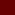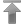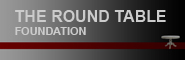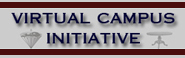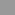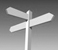Contenido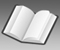Datos# Glosario

 a b c d e f g h i j k l m n o p q r s t u v w x y z

### J

Joule, symbol J. 1 J is equal to the energy expended (or work done) in applying a force of one Newton through a distance of one meter (1 newton meter or N × m), or in passing an electric current of one ampere through a resistance of one ohm for one second.